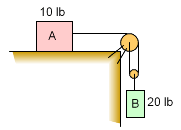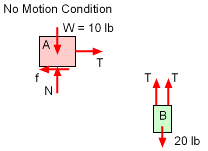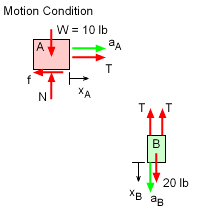Ch 2. Particle Force and Acceleration Multimedia Engineering Dynamics Rect.Coord. Normal/Tang. Coord. PolarCoord. Orbital Mechanics Computational Mechanics
 Chapter - Particle - 1. General Motion 2. Force & Accel. 3. Energy 4. Momentum - Rigid Body - 5. General Motion 6. Force & Accel. 7. Energy 8. Momentum 9. 3-D Motion 10. Vibrations Appendix Basic Math Units Basic Equations Sections Search eBooks Dynamics Fluids Math Mechanics Statics Thermodynamics Author(s): Kurt Gramoll ©Kurt GramollDYNAMICS - EXAMPLEDouble Block Motion Example Two different blocks are connected through a pull system and released. What is tension in the cable? The coefficient of static and kinetic friction between block A and the ground is 0.4 and 0.25, respectively. Assume the pulley is massless and frictionless. SolutionCheck if Blocks are in Static Equilibrium Two actions can take place with this system. First, the coefficient of static friction is sufficient to keep the block from moving (No Motion Condition). This situation will occur when      f ≥ T The tension in the cable is simply half the weight of block B, or 10 lb. The friction force, f, will be μN, or 0.4(10 lb) = 4 lb. Thus, f is not larger than the tension, and the blocks will move. This is not surprising since this is a dynamics course.Block Motion Since the blocks will move, each will have an acceleration that directly affects the cable tension. Free-body diagrams with acceleration vectors for each block helps organize all actions affecting the blocks. Summing forces and accelerations give      Block A:  T - f = aAmA      Block B:  20 - 2T = aBmB The friction is simply N = 0.25(10) = 2.5 lb using the kinetic coefficient of friction. But the acceleration terms need to be related to reduce the unknowns to just two. The pulley effectively reduces the motion of B to half the motion of A, or in equation form,       xB = 0.5xA This can be integrated twice to give,      aB = 0.5aA Substituting known information into basic equations give,      Block A:  T - 2.5 = aA (10/g)      Block B:  20 - 2T = 0.5aA (20/g) Combining,      20 - 2T = 0.5 [(T - 2.5) (g/10)] (20/g)      20 - 2T = T - 2.5      22.5 = 3T Solving gives, T = 7.5 lb It is interesting to note, if there was no friction, the equation becomes,      20 - 2T = 0.5 [(T - 0) (g/10)] (20/g)      20 = 3T or Tno friction = 6.667 lb

Practice Homework and Test problems now available in the 'Eng Dynamics' mobile app
Includes over 400 problems with complete detailed solutions.
Available now at the Google Play Store and Apple App Store.